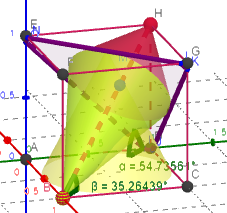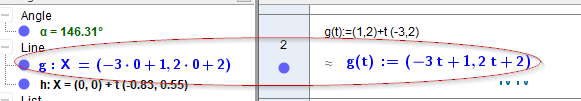# minimum command V6.x classic online app

hawe shared this problem 1 year ago
New

See

(local Apps 5.x, 6.x)

r (min-command) is set to the shortest distance to planes z=0 or z=1

set slider k=0.65Online Version seems not to min distance - please try

but comming back over night the online version has come back to bussines and is working fine.

after reloading app the problem coming back1

```r=Min({sqrt((M-Intersect(g,z=0))^(2)), sqrt((M-Intersect(g,z=a))^(2))})
```

Try splitting that up into smaller "chunks"1

Also Distance() command might be useful?1

A workaround was to put it in a if-clause...

but curios that only the online app is affected.

BTW: just now found1

I think the reason of this inconsistency is intercept(ion) with plane z=0 return a R^2 point instead of R^3 point...

I do not use intersept command - compute a CAS formula1

Makes sense, you can do

```B=(x(A),y(A),0)
```

to convert if necessary